# Adding And Subtracting Standard Form Worksheet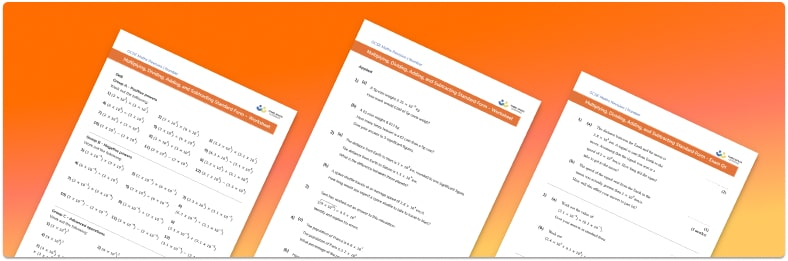• This field is for validation purposes and should be left unchanged.

You can unsubscribe at any time (each email we send will contain an easy way to unsubscribe). To find out more about how we use your data, see our privacy policy.

• Section 1 of the Adding and Subtracting Standard Form worksheet contains 12 skills-based Adding and Subtracting Standard Form questions, in 3 groups to support differentiation
• Section 2 contains 4 applied Adding and Subtracting Standard Form questions with a mix of worded problems and deeper problem solving questions
• Section 3 contains 4 foundation and higher level GCSE exam style Adding and Subtracting Standard Form questions
• Answers and a mark scheme for all Adding and Subtracting Standard Form questions
• Follows variation theory with plenty of opportunities for students to work independently at their own level
• All questions created by fully qualified expert secondary maths teachers
• Suitable for GCSE maths revision for AQA, OCR and Edexcel exam boards

### Adding and subtracting standard form at a glance

Standard form, or standard index form, is a simple way of writing very large numbers or very small numbers. To convert numbers to standard form, we write them as decimals between 1 and 10, multiplied by a power of 10.  We call numbers that are not written in standard form ordinary numbers.

If we are adding or subtracting two standard form numbers with different powers of 10, the place value of the numbers written in the decimals will be different so we cannot add or subtract them directly. Therefore, when adding and subtracting numbers in standard form we first convert the numbers back to ordinary numbers. Once we have added or subtracted them, we can convert the answer back into standard form.

Alternatively we can make the both powers of 10 the same by adjusting the number. Once the powers of 10 are the same we can then add or subtract the numbers, taking care to ensure the final answer is in standard form.

Looking forward, students can then progress to additional number worksheets, for example aor aFor more teaching and learning support on Number our GCSE maths lessons provide step by step support for all GCSE maths concepts.

## Related worksheets

Converting To And From Standard Form Worksheet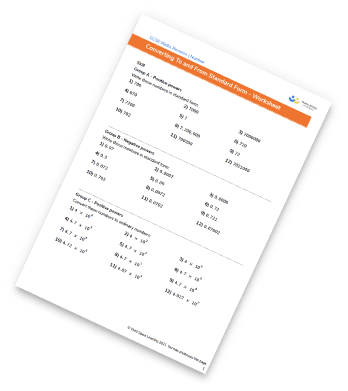Standard Form Worksheet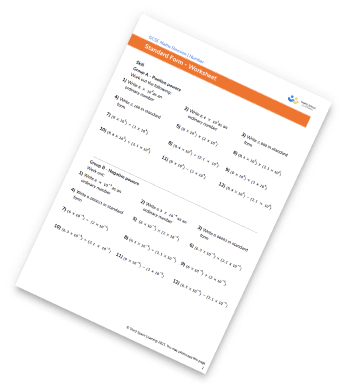Rational And Irrational Numbers Worksheet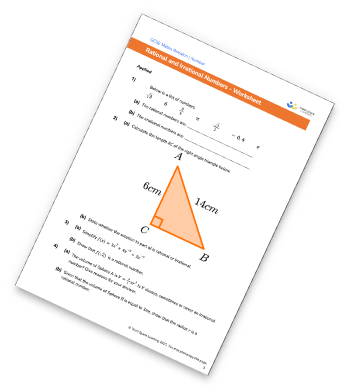Surds Worksheet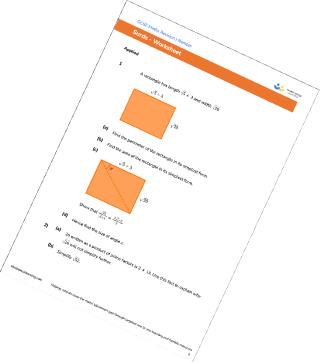## Do you have KS4 students who need more focused attention to succeed at GCSE?There will be students in your class who require individual attention to help them succeed in their maths GCSEs. In a class of 30, it’s not always easy to provide.

Help your students feel confident with exam-style questions and the strategies they’ll need to answer them correctly with our dedicated GCSE maths revision programme.

Lessons are selected to provide support where each student needs it most, and specially-trained GCSE maths tutors adapt the pitch and pace of each lesson. This ensures a personalised revision programme that raises grades and boosts confidence.

Find out more